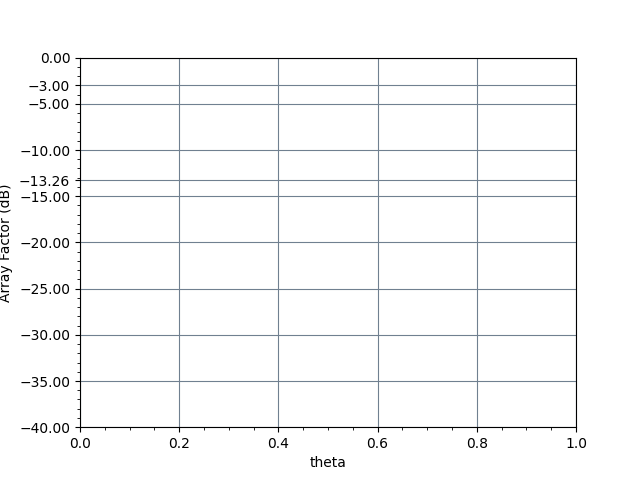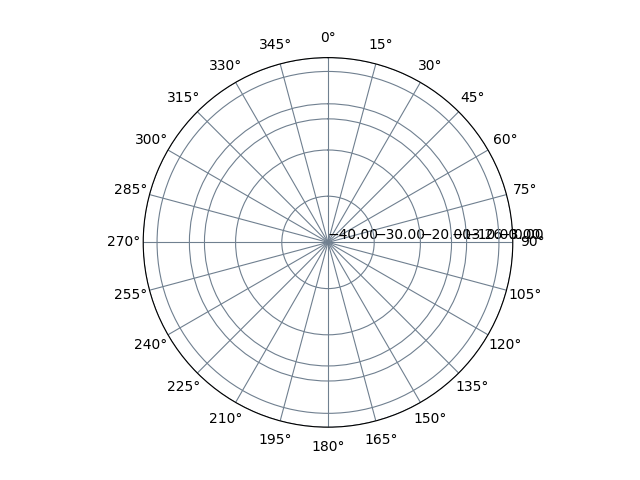# Radiation Pattern of Uniform Linear Antenna Array

 No. of Elements N Element Spacing in Wavelength $$\lambda$$ d Phase Shift in degree $$\beta$$ Erase Graph Erase GraphOverlay Graph Graph Legend

 Normalized radiation pattern in rectangular plot YZ Cut Normalized radiation pattern in polar plot YZ Cut## Formula

$$AF = \sum_{n=1}^{N}e^{j(n-1)(kdcos\theta +\beta )}$$
 Where: The angular wavenumber $$k=\frac{2\pi }{\lambda }$$ expresses the number of radians in a unit of distance N = number of antenna elements d = distance between the antenna elements $$\beta$$ = relative phase shift
← RF Theory